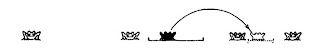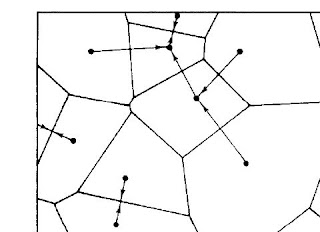## Wednesday, April 28, 2010

### Mathematical Explanation in the NYRB

In his recent review of Dawkins' Oxford Book of Modern Science Writing Jeremy Bernstein characterizes one entry as follows:
W.D. Hamilton’s mathematical explanation of the tendency of animals to cluster when attacked by predators.
The article in question is "Geometry for the Selfish Herd", Journal of Theoretical Biology 31 (1971): 295-311. (Online here.) Given the ongoing worries about the existence and nature of mathematical explanations in science, it is worth asking what led Bernstein to characterize this explanation as mathematical?

The article summarizes two models of predation which are used to support the conclusion that the avoidance of predators "is an important factor in the gregarious tendencies of a very wide variety of animals" (p. 298). The first model considers a circular pond where frogs, the prey, are randomly scattered on the edge. The predator, a single snake, comes to the surface of the pond and strikes whichever frog is nearest. Hamilton introduces a notion of the domain of danger of a frog which is the part of the pond edge which would lead to the frog being attacked. Hamilton points out that the frogs can reduce their domains of danger by jumping together. In this diagram the black frog jumps between two other frogs:So, "selfish avoidance of a predator can lead to aggregation."

In the slightly more realistic two-dimensional case Hamilton generalizes his domains of danger to polygons whose sides result from bisecting the lines which connect the prey:Hamilton notes that it is not known what the general best strategy is here for a prey organism to minimize its domain of danger, but gives rough estimates to justify the conclusion that moving towards ones nearest neighbor is appropriate. This is motivated in part by the claim that "Since the average number of sides is six and triangles are rare (...), it must be a generally useful rule for a cow to approach its nearest neighbor."

So, we can explain the observed aggregation behavior using the ordinary notion of fitness and an appeal to natural selection. What is the mathematics doing here and why might we have some sort of specifically mathematical explanation? My suggestion is that the mathematical claim that strategy X minimizes (or reliably lowers) the domain of danger is a crucial part of the account. Believing this claim and seeing its relevance to the aggregation behavior is essential to having this explanation. Furthermore, this seems like a very good explanation. What implications this has for our mathematical beliefs remains, of course, a subject for debate.